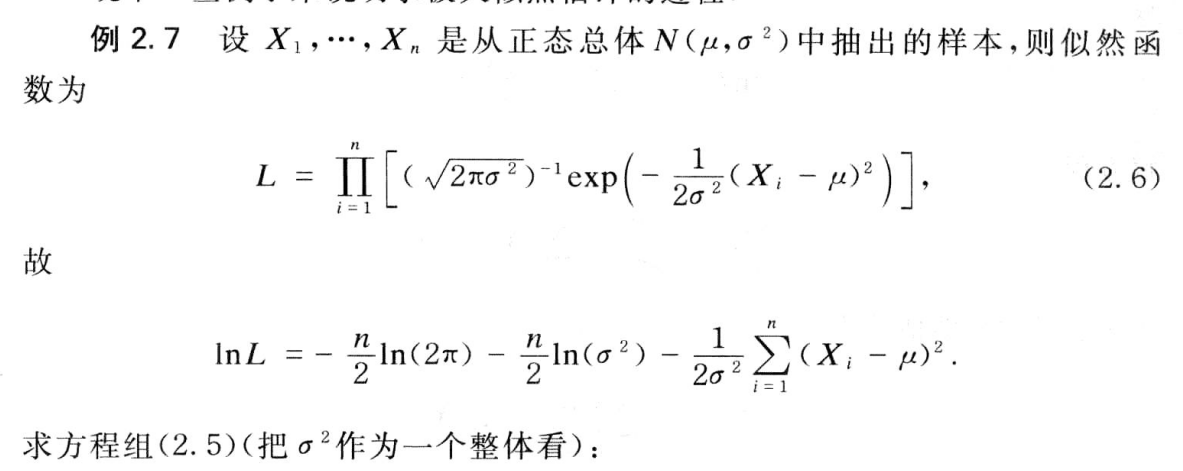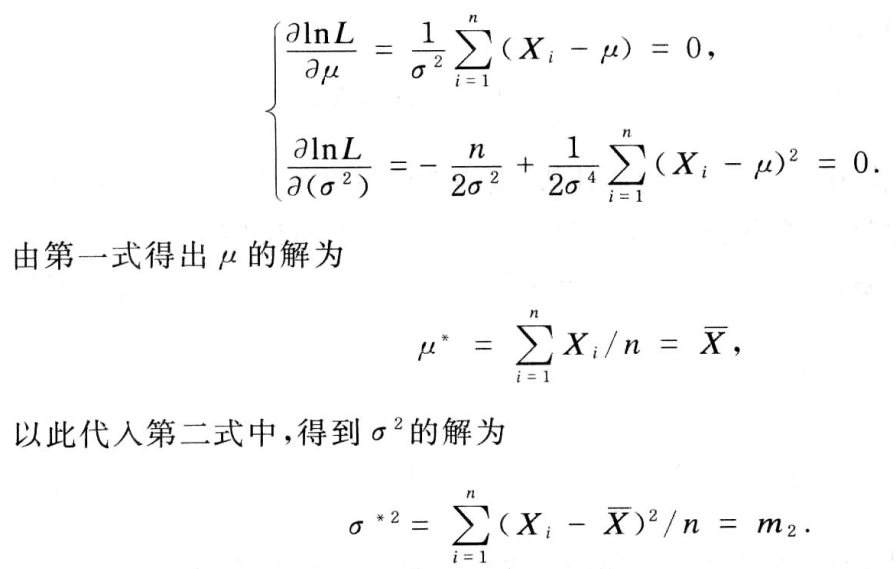# 极大似然法估计

hit2015spring $\frac{hit2015spring}{晨凫追风}$

f(x1;θ1,θ2,,θk)f(x2;θ1,θ2,,θk)f(xn;θ1,θ2,,θk) $f(x_1;\theta_1,\theta_2,\cdots,\theta_k)f(x_2;\theta_1,\theta_2,\cdots,\theta_k)\cdots f(x_n;\theta_1,\theta_2,\cdots,\theta_k)$

1 $1^\circ$当我们固定 θ1,,θk $\theta_1,\cdots,\theta_k$时，看做是 x1,,xn $x_1,\cdots,x_n$的函数， L $L$是一个概率密度函数或者概率函数。

2 $2^\circ$当我们固定 x1,,xn $x_1,\cdots,x_n$时， L(X1,,Xn;θ1,,θk) $L(X_1,\cdots,X_n;\theta_1,\cdots,\theta_k)$看做是 θ1,,θk $\theta_1,\cdots,\theta_k$的函数， L $L$是一个似然估计，这个函数在一个固定的观察结果x1,,xn$x_1,\cdots,x_n$的取值下，参数值 θ1,,θk $\theta_1,\cdots,\theta_k$可以看成是导致这个结果出现的原因，因为出现了周芷若接受糖果的事了，所以我们就让这件事情发生的概率最大，所以就叫张无忌去送糖果。张无忌就是那个 θ⃗ 1,,θ⃗ k $\vec\theta_1,\cdots,\vec\theta_k$。当然这里面的 θ1,,θk $\theta_1,\cdots,\theta_k$是有一定的值的（并不是任何值都可以），并不是随便一个人送糖果都可以的对吧。这里还包含了贝叶斯学派和频率主义学派两家的观点问题。频率主义学派认为参数是虽然是未知的，但是它是一个客观存在的固定值，因此可以通过优化似然函数等一些准则来确定参数值；但是贝叶斯学派认为参数是未观察到的随机变量，其本身也可以有分布，因此可以假定参数服从一个先验分布，然后基于观测到的数据来计算参数的后验分布。上面的估计方法就是传统的频率主义学派所认为的观点，就是一个事件的概率分布参数是存在的，我们需要优化似然函数这样的函数来求解得到参数。既然能发生，说明它出现的概率就是大，就像能考到清北的孩子优秀的概率肯定大于一般高校的孩子（一般这样认为）。

L(X1,,Xn;θ1,,θk)=maxθ1,,θkL(Y1,,Yn;θ1,,θk) $L(X_1,\cdots,X_n;\theta_1^*,\cdots,\theta_k^*) = \max\limits_{\theta_1,\cdots,\theta_k} L(Y_1,\cdots,Y_n;\theta_1,\cdots,\theta_k)$

lnL=i=1nlnf(Xi;θ1,,θk) $\ln L=\sum\limits_{i=1}^n \ln f(X_i;\theta_1,\cdots , \theta_k)$

lnLθi=0 $\frac{\partial \ln L}{\partial \theta_i}=0$11-18941
07-3125112-17183
03-103422
08-10
03-24
06-10
01-04
10-25